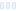Essential Formula

Multivitamin - Meijer

Main info:

Essential Formula
Multivitamin - Meijer
1 tablet
0 Calories
0 g
0 g
0 g

0 g
0 mg
0 g
0 mg
0 g
0 g

Percent calories from...
Nutrition Facts
For a Serving Size of (g)
How many calories are in Essential Formula? Amount of calories in Essential Formula: Calories Calories from Fat (%)
% Daily Value *
How much fat is in Essential Formula? Amount of fat in Essential Formula: Total Fat
How much sodium is in Essential Formula? Amount of sodium in Essential Formula: Sodium
How many carbs are in Essential Formula? Amount of carbs in Essential Formula: Carbohydrates
How many net carbs are in Essential Formula? Amount of net carbs in Essential Formula: Net carbs
How much fiber is in Essential Formula? Amount of fiber in Essential Formula: Fiber
How much protein is in Essential Formula? Amount of protein in Essential Formula: Protein
Vitamins and minerals
How much Vitamin A is in Essential Formula? Amount of Vitamin A in Essential Formula: Vitamin A
How much Vitamin C is in Essential Formula? Amount of Vitamin C in Essential Formula: Vitamin C
How much Calcium is in Essential Formula? Amount of Calcium in Essential Formula: Calcium
How much Iron is in Essential Formula? Amount of Iron in Essential Formula: Iron
Fatty acids
Amino acids
* The Percent Daily Values are based on a 2,000 calorie diet, so your values may change depending on your calorie needs.Loading similar foods...
Note: Any items purchased after clicking our Amazon buttons will give us a little referral bonus. If you do click them, thank you!Be cool

- Zen orangeI never skip arm day

- Buff broccoli